# Torelli theorems

Theorems stating that the Hodge structure (period matrix) in the cohomology spaces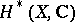of an algebraic or Kähler varietycompletely characterizes the polarized Jacobi variety of.

The classical Torelli theorem relates to the case of curves (see , ) and states that a curve is defined up to an isomorphism by its periods. Letbe a curve of genus, let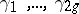be a basis of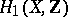, letbe a basis of the Abelian differentials (cf. Abelian differential) and let the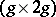-matrixbe the period matrix, where. The intersection of cyclesdefines a skew-symmetric bilinear formin. Letandbe two curves. If basesandcan be chosen with respect to which the period matricesand the intersection matricesof the curves are the same, thenand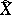are isomorphic. In other words, if the canonically polarized Jacobians of the curvesandare isomorphic, then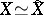.

Letbe a projective variety (or, more generally, a compact Kähler manifold), and let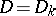be the Griffiths variety associated with the primitive cohomology spaces(see Period mapping). Thencontains the period matrices of primitive-forms on all varieties homeomorphic to. The periods depend on the choice of the isomorphism ofinto a fixed space. There is a naturally defined groupof analytic automorphisms ofsuch thatis an analytic space anddetermines a unique point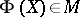. In this situation,is called the modular space or the moduli space of Hodge structures.

The global Torelli problem consists in the elucidation of the question whether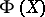uniquely determinesup to an isomorphism. In the case of an affirmative solution, the problem corresponds to the statement of the so-called (generalized) Torelli theorem. Torelli's theorem holds trivially for Abelian varieties in the case of-forms and in the case of-forms (see ). Essentially, the only non-trivial case of a solution of the global Torelli problem (1984) is the case of a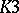-surface. The Torelli theorem has also been generalized to the case of Kähler-surfaces.

The local Torelli problem consists in solving the question when the Hodge structures on the cohomology spaces separate points in the local moduli space (the Kuranishi space) for a variety. Let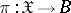be a family of polarized algebraic varieties,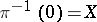, and letbe the Griffiths variety associated with the periods of primitive-forms on. The period mapping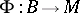associates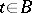with the period matrix of-forms on. This mapping is holomorphic; the corresponding tangent mapping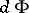has been calculated (see ). The local Torelli problem is equivalent to the question: When isan imbedding? By considering the mapping dual toone obtains a cohomological criterion for the validity of the local Torelli theorem: If the mappingis an epimorphism, then the periods of the-forms give local moduli for. The local Torelli theorem for curves is equivalent to the fact that quadratic differentials are generated by Abelian differentials. Noether's theorem states that this is true if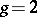or if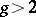andis not hyper-elliptic. The local Torelli theorem clearly holds in the caseif the canonical class is trivial. Such varieties include the Abelian varieties, hypersurfaces of degreein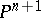and-surfaces. The validity of the local Torelli theorem has been established for various classes of higher-dimensional varieties. For non-singular hypersurfaces of degreeinit has been proved that the period mapping is an imbedding at a generic point except for the case,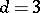and, possibly, the cases:divides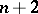,and, orand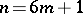(see ).